Current Electricity - Lesson 1 - Electric Potential Difference

# Electric Potential Difference

In the previous section of Lesson 1, the concept of electric potential was introduced. Electric potential is a location-dependent quantity that expresses the amount of potential energy per unit of charge at a specified location. When a Coulomb of charge (or any given amount of charge) possesses a relatively large quantity of potential energy at a given location, then that location is said to be a location of high electric potential. And similarly, if a Coulomb of charge (or any given amount of charge) possesses a relatively small quantity of potential energy at a given location, then that location is said to be a location of low electric potential. As we begin to apply our concepts of potential energy and electric potential to circuits, we will begin to refer to the difference in electric potential between two points. This part of Lesson 1 will be devoted to an understanding of electric potential difference and its application to the movement of charge in electric circuits.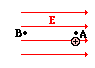Consider the task of moving a positive test charge within a uniform electric field from location A to location B as shown in the diagram at the right. In moving the charge against the electric field from location A to location B, work will have to be done on the charge by an external force. The work done on the charge changes its potential energy to a higher value; and the amount of work that is done is equal to the change in the potential energy. As a result of this change in potential energy, there is also a difference in electric potential between locations A and B. This difference in electric potential is represented by the symbol ΔV and is formally referred to as the electric potential difference. By definition, the electric potential difference is the difference in electric potential (V) between the final and the initial location when work is done upon a charge to change its potential energy. In equation form, the electric potential difference is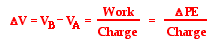The standard metric unit on electric potential difference is the volt, abbreviated V and named in honor of Alessandro Volta. One Volt is equivalent to one Joule per Coulomb. If the electric potential difference between two locations is 1 volt, then one Coulomb of charge will gain 1 joule of potential energy when moved between those two locations. If the electric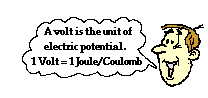potential difference between two locations is 3 volts, then one coulomb of charge will gain 3 joules of potential energy when moved between those two locations. And finally, if the electric potential difference between two locations is 12 volts, then one coulomb of charge will gain 12 joules of potential energy when moved between those two locations. Because electric potential difference is expressed in units of volts, it is sometimes referred to as the voltage.

### Electric Potential Difference and Simple Circuits

Electric circuits, as we shall see, are all about the movement of charge between varying locations and the corresponding loss and gain of energy that accompanies this movement. In the previous part of Lesson 1, the concept of electric potential was applied to a simple battery-powered electric circuit. In that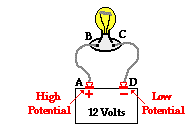discussion, it was explained that work must be done on a positive test charge to move it through the cells from the negative terminal to the positive terminal. This work would increase the potential energy of the charge and thus increase its electric potential. As the positive test charge moves through the external circuit from the positive terminal to the negative terminal, it decreases its electric potential energy and thus is at low potential by the time it returns to the negative terminal. If a 12 volt battery is used in the circuit, then every coulomb of charge is gaining 12 joules of potential energy as it moves through the battery. And similarly, every coulomb of charge loses 12 joules of electric potential energy as it passes through the external circuit. The loss of this electric potential energy in the external circuit results in a gain in light energy, thermal energy and other forms of non-electrical energy.

With a clear understanding of electric potential difference, the role of an electrochemical cell or collection of cells (i.e., a battery) in a simple circuit can be correctly understood. The cells simply supply the energy to do work upon the charge to move it from the negative terminal to the positive terminal. By providing energy to the charge, the cell is capable of maintaining an electric potential difference across the two ends of the external circuit. Once the charge has reached the high potential terminal, it will naturally flow through the wires to the low potential terminal. The movement of charge through an electric circuit is analogous to the movement of water at a water park or the movement of roller coaster cars at an amusement park. In each analogy, work must be done on the water or the roller coaster cars to move it from a location of low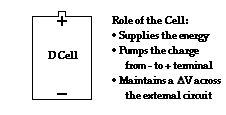gravitational potential to a location of high gravitational potential. Once the water or the roller coaster cars reach high gravitational potential, they naturally move downward back to the low potential location. For a water ride or a roller coaster ride, the task of lifting the water or coaster cars to high potential requires energy. The energy is supplied by a motor-driven water pump or a motor-driven chain. In a battery-powered electric circuit, the cells serve the role of the charge pump to supply energy to the charge to lift it from the low potential position through the cell to the high potential position.

It is often convenient to speak of an electric circuit such as the simple circuit discussed here as having two parts - an internal circuit and an external circuit. The internal circuit is the part of the circuit where energy is being supplied to the charge. For the simple battery-powered circuit that we have been referring to, the portion of the circuit containing the electrochemical cells is the internal circuit. The external circuit is the part of the circuit where charge is moving outside the cells through the wires on its path from the high potential terminal to the low potential terminal. The movement of charge through the internal circuit requires energy since it is an uphill movement in a direction that is against the electric field. The movement of charge through the external circuit is natural since it is a movement in the direction of the electric field. When at the positive terminal of an electrochemical cell, a positive test charge is at a high electric pressure in the same manner that water at a water park is at a high water pressure after being pumped to the top of a water slide. Being under high electric pressure, a positive test charge spontaneously and naturally moves through the external circuit to the low pressure, low potential location.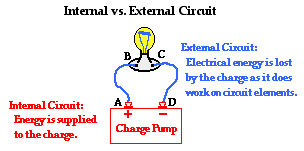As a positive test charge moves through the external circuit, it encounters a variety of types of circuit elements. Each circuit element serves as an energy-transforming device. Light bulbs, motors, and heating elements (such as in toasters and hair dryers) are examples of energy-transforming devices. In each of these devices, the electrical potential energy of the charge is transformed into other useful (and non-useful) forms. For instance, in a light bulb, the electric potential energy of the charge is transformed into light energy (a useful form) and thermal energy (a non-useful form). The moving charge is doing work upon the light bulb to produce two different forms of energy. By doing so, the moving charge is losing its electric potential energy. Upon leaving the circuit element, the charge is less energized. The location just prior to entering the light bulb (or any circuit element) is a high electric potential location; and the location just after leaving the light bulb (or any circuit element) is a low electric potential location. Referring to the diagram above, locations A and B are high potential locations and locations C and D are low potential locations. The loss in electric potential while passing through a circuit element is often referred to as a voltage drop. By the time that the positive test charge has returned to the negative terminal, it is at 0 volts and is ready to be re-energized and pumped back up to the high voltage, positive terminal.

### Electric Potential Diagrams

An electric potential diagram is a convenient tool for representing the electric potential differences between various locations in an electric circuit. Two simple circuits and their corresponding electric potential diagrams are shown below.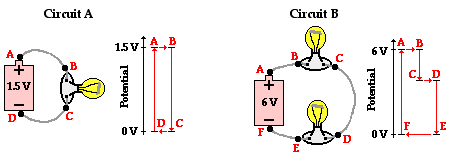In Circuit A, there is a 1.5-volt D-cell and a single light bulb. In Circuit B, there is a 6-volt battery (four 1.5-volt D-cells) and two light bulbs. In each case, the negative terminal of the battery is the 0 volt location. The positive terminal of the battery has an electric potential that is equal to the voltage rating of the battery. The battery energizes the charge to pump it from the low voltage terminal to the high voltage terminal. By so doing the battery establishes an electric potential difference across the two ends of the external circuit. Being under electric pressure, the charge will now move through the external circuit. As its electric potential energy is transformed into light energy and heat energy at the light bulb locations, the charge decreases its electric potential. The total voltage drop across the external circuit equals the battery voltage as the charge moves from the positive terminal back to 0 volts at the negative terminal. In the case of Circuit B, there are two voltage drops in the external circuit, one for each light bulb. While the amount of voltage drop in an individual bulb depends upon various factors (to be discussed later), the cumulative amount of drop must equal the 6 volts gained when moving through the battery.

### Investigate!

The electrical potential difference across the two inserts of a household electrical outlet varies with the country. Use the Household Voltages widget below to find out the household voltage values for various countries (e.g., United States, Canada, Japan, China, South Africa, etc.).

### Check Your Understanding

1. Moving an electron within an electric field would change the ____ the electron.

a. mass of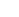b. amount of charge onc. potential energy of

2. If an electrical circuit were analogous to a water circuit at a water park, then the battery voltage would be comparable to _____.

a. the rate at which water flows through the circuit

b. the speed at which water flows through the circuit

c. the distance that water flows through the circuit

d. the water pressure between the top and bottom of the circuit

e. the hindrance caused by obstacles in the path of the moving water

3. If the electrical circuit in your Walkman were analogous to a water circuit at a water park, then the battery would be comparable to _____.

a. the people that slide from the elevated positions to the ground

b. the obstacles that stand in the path of the moving water

c. the pump that moves water from the ground to the elevated positions

d. the pipes through which water flows

e. the distance that water flows through the circuit

4. Which of the following is true about the electrical circuit in your flashlight?

a. Charge moves around the circuit very fast - nearly as fast as the speed of light.

b. The battery supplies the charge (electrons) that moves through the wires.

c. The battery supplies the charge (protons) that moves through the wires.

d. The charge becomes used up as it passes through the light bulb.

e. The battery supplies energy that raises charge from low to high voltage.

f. ... nonsense! None of these are true.

5. If a battery provides a high voltage, it can ____.

a. do a lot of work over the course of its lifetime

b. do a lot of work on each charge it encounters

c. push a lot of charge through a circuit

d. last a long time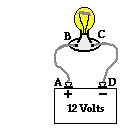The diagram below at the right shows a light bulb connected by wires to the + and - terminals of a car battery. Use the diagram in answering the next four questions.

6. Compared to point D, point A is _____ electric potential.

a. 12 V higher in

b. 12 V lower in

c. exactly the same

d. ... impossible to tell

7. The electric potential energy of a charge is zero at point _____.

8. Energy is required to force a positive test charge to move ___.

a. through the wire from point A to point B

b. through the light bulb from point B to point C

c. through the wire from point C to point D

d. through the battery from point D to point A

9. The energy required to move +2 C of charge between points D and A is ____ J.

a. 0.167b. 2.0c. 6.0d. 12e. 24

10. The following circuit consists of a D-cell and a light bulb. Use >, <, and = symbols to compare the electric potential at A to B and at C to D. Indicate whether the devices add energy to or remove energy from the charge.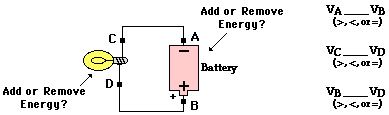11. Use your understanding of the mathematical relationship between work, potential energy, charge and electric potential difference to complete the following statements:

a. A 9-volt battery will increase the potential energy of 1 coulomb of charge by ____ joules.

b. A 9-volt battery will increase the potential energy of 2 coulombs of charge by ____ joules.

c. A 9-volt battery will increase the potential energy of 0.5 coulombs of charge by ____ joules.

d. A ___-volt battery will increase the potential energy of 3 coulombs of charge by 18 joules.

e. A ___-volt battery will increase the potential energy of 2 coulombs of charge by 3 joules.

f. A 1.5-volt battery will increase the potential energy of ____ coulombs of charge by 0.75 joules.

g. A 12-volt battery will increase the potential energy of ____ coulombs of charge by 6 joules.